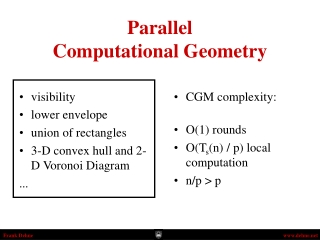DownloadDownload PresentationParallel Computational Geometry

# Parallel Computational Geometry

Download Presentation## Parallel Computational Geometry

- - - - - - - - - - - - - - - - - - - - - - - - - - - E N D - - - - - - - - - - - - - - - - - - - - - - - - - - -
##### Presentation Transcript

1. visibility lower envelope union of rectangles 3-D convex hull and 2-D Voronoi Diagram ... CGM complexity: O(1) rounds O(Ts(n) / p) local computation n/p > p ParallelComputational Geometry

2. Lower Envelope • Given: A set S of n non-intersecting line segments S

3. Lower Envelope • Given: A set S of n non-intersecting line segments S LE(S) • Task: Compute the lower envelope LE(S)

4. Lower Envelope • Goal: O(1) rounds • Problem: Each processor “sees” only O(n/p) line segments

5. Lower Envelope proc. 1 proc. 2 proc. 3 proc. 4 S1 S2 S3 S4 S = S1S2S3S4

6. Lower Envelope proc. 1 proc. 2 proc. 3 proc. 4 S1 S2 S3 S4 S = S1S2S3S4 • Step 1: Each proc. Pi computes locally LE(Si)

7. Lower Envelope proc. 1 proc. 2 proc. 3 proc. 4 S1 S2 S3 S4 S = S1S2S3S4 • Step 1: Each proc. Pi computes locally LE(Si) proc. 1 proc. 2 proc. 3 proc. 4

8. Lower Envelope • Step 2: Globally sort [i=1..pLE(Si)] by x-coord. of right endpoint

9. Lower Envelope • Step 2: Globally sort [i=1..pLE(Si)] by x-coord. of right endpoint proc. 1 proc. 2 proc. 3 proc. 4 V1 V2 V3 V4

10. Lower Envelope • Step 3: Each Pi determines Li and broadcasts Li to all other Pj (one h-relation) proc. 1 proc. 2 proc. 3 proc. 4 V1 V2 V3 V4 L1 L2 L3 L4

11. Lower Envelope • Step 4: Each Pi scans LE(Si) and sends the segment intersecting Lj to Pj for j=1..p (one h-relation) proc. 1 proc. 2 proc. 3 proc. 4 R1 R2 R3 R4 V1 V2 V3 V4 L1 L2 L3 L4

12. Lower Envelope • Step 5: Each Pi computes LE(Vi Ri) proc. 1 proc. 2 proc. 3 proc. 4 R1 R2 R3 R4 V1 V2 V3 V4 L1 L2 L3 L4

13. Lower Envelope